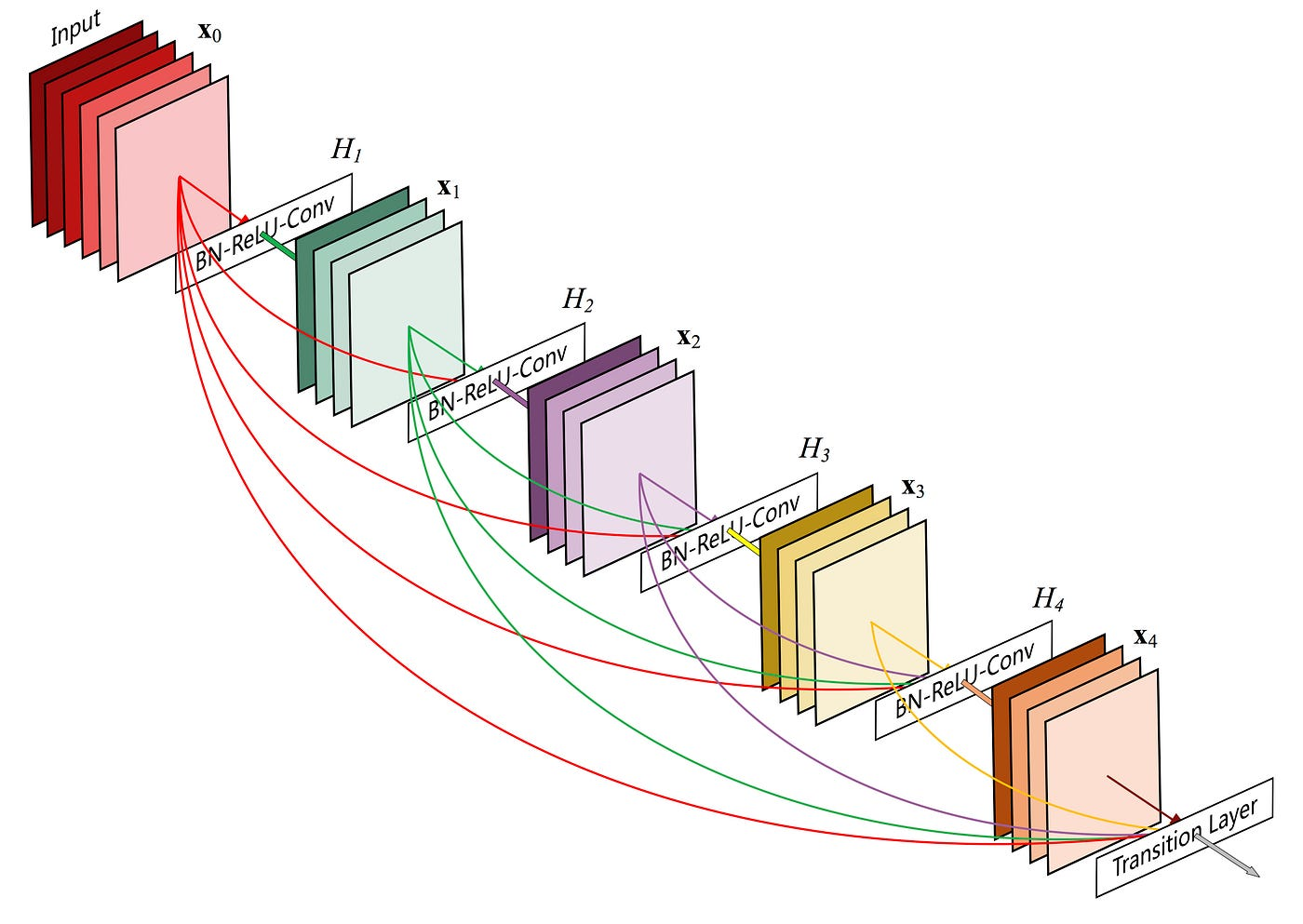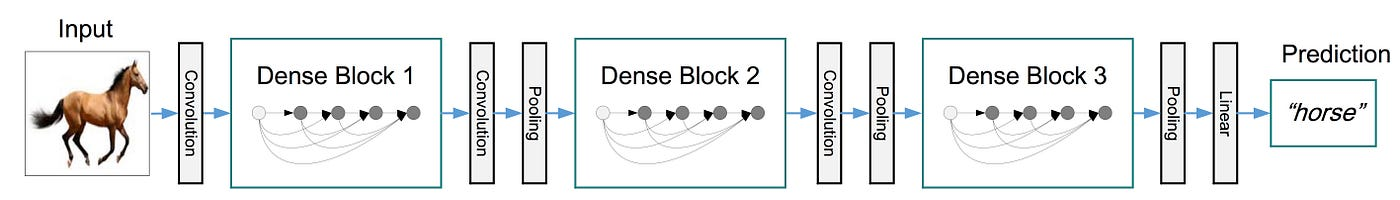# Image Classification using pre-trained DenseNet model in PyTorch | by Bhimraj YadavFigure 1: A 5-layer dense block with a growth rate of k = 4Figure 2: A deep DenseNet with three dense blocks.

# 6 Simple Steps to be followed:

1. Install and import required libraries
2. Download an example Image and ImageNet output classes and load them
3. Apply transformations to the image
4. Load DenseNet model
5. Get the final result/output.
6. Plot the result
`# Optional Part: To create an environmentpython -m venv venvsource venv/bin/activate# install these librariespip install torch torchvision Pillow matplotlib`
`import torchfrom PIL import Imagefrom torchvision import transforms,models    import matplotlib.pyplot as plt`
`# Download an example image from the pytorch website!wget "https://github.com/pytorch/hub/raw/master/images/dog.jpg" -O "dog.jpg"# Download ImageNet labels!wget https://raw.githubusercontent.com/pytorch/hub/master/imagenet_classes.txt# Read the image classeswith open("imagenet_classes.txt", "r") as f:    img_classes = [s.strip() for s in f.readlines()]print("Total Image Classes :{}".format(len(img_classes)))print(img_classes[:5]) #displaying first five classes# Printed OutputTotal Image Classes :1000 ['tench', 'goldfish', 'great white shark', 'tiger shark', 'hammerhead']`
`# transforming input imagefilename = "dog.jpg"input_image = Image.open(filename)preprocess = transforms.Compose([          transforms.Resize(256),          transforms.CenterCrop(224),          transforms.ToTensor(),          transforms.Normalize(mean=[0.485, 0.456, 0.406],                                std=[0.229, 0.224, 0.225]),])input_tensor = preprocess(input_image)# create a mini-batch as expected by the modelinput_batch = input_tensor.unsqueeze(0) `
`# modelmodel = models.densenet121(pretrained=True)model.eval()  # sets the model in evaluation mode# For more info about evaluation:# https://stackoverflow.com/questions/60018578/what-does-model-eval-do-in-pytorch# move the input and model to GPU for speed if availableif torch.cuda.is_available():   input_batch = input_batch.to('cuda')   model.to('cuda')`
`with torch.no_grad():   output = model(input_batch)# Tensor of shape 1000, with confidence scores over Imagenet's 1000 classes# The output has unnormalized scores. To get probabilities, you can run a softmax on it.probabilities = torch.nn.functional.softmax(output, dim=0)# Show top categories per imagetop5_prob, top5_catid = torch.topk(probabilities, 5)for id,prob in zip(top5_catid,top5_prob):  print(f'Image class: {img_classes[id]}\t----- {prob * 100:.4f} %')# Printed OutputImage class: Samoyed ----- 86.7997 % Image class: white wolf ----- 4.3434 % Image class: keeshond ----- 2.5546 % Image class: Arctic fox ----- 2.3647 % Image class: Pomeranian ----- 1.7686 %`
`classes = [img_classes[id] for id in top5_catid]probabilities = [prob * 100 for prob in top5_prob]# creating the bar plotfig, (ax1,ax2) = plt.subplots(1,2,figsize=(24, 8))ax1.set_title('Input Image of Dog')ax1.imshow(input_image)ax2.bar(classes, probabilities)ax2.set_xlabel("Top 5 Classes")ax2.set_ylabel("Probabilities")ax2.set_title("Image Classification")plt.show()`